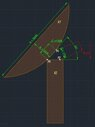# Required force to hold or pull inclined object downwards.

AK93
Thread moved from a technical forum to the schoolwork forums
Can somebody help me to solve this problem and guide? I need to calculate minimum force (F) (Newtons or kilograms) is required to pull down object (K1) to (K2), or to hold it in position. Angle between (K1) and (K2) = 50 degrees. Using mounting points (P1) and (P2). (K1) mass = 3.5 ton, (A) Length = 9 meters, (B) Height = 3.5 meters. Mounting points (P1) and (P3 is on a hinge) distance from center = 75centimeters, (C) = 1.3 meters in total. Height from point (P1) to (P2) is 1.7 meters.

#### Attachments

•eaWNr.jpg
34.4 KB · Views: 23

Welcome to PF.

First, you will need to identify the position of the centre of mass of k1.

But gravity will not be your biggest problem. The wind will be more important. You must model k1 for wind loading at different elevation angles and wind directions. Is this structure in a region subjected to hurricane force winds?

•AK93
Homework Helper
Gold Member
Welcome!
What have you calculated so far?
Could you show us your work?

Where will the pulling force be applied?
At what point will the round upper surface hit the flat lower surface?

•AK93
AK93
Welcome!
What have you calculated so far?
Could you show us your work?

Where will the pulling force be applied?
At what point will the round upper surface hit the flat lower surface?
Thanks for the nice greeting,
I haven't done any calculations. Thats why Iam asking for a guide and help, how should I calculate the forces. P1 and P2 must meet, so K1 will be looking upwards. Biggest question, how much force is needed to hold it in position or pill it downwards so K1 wouldn't flip to the left side. I can find the center of the K1, what else should be done to calculate this?

Once K1 is looking upwards, the centre of mass will be above K2, between the hinge and point P2. K1 will then be resting on K2, with P1 pressed against P2. Force will not be needed to hold K1 down.

The mass of K1, and the position of the centre of mass of K1 are needed.

The line from the hinge pin, P3, to the centre of mass forms a radial arm. When that arm points directly upwards, no torque will be needed to balance K1. Either side of that balance point, an angle and mass dependent force will be required.

We can explain to you what is important, but you must employ a qualified mechanical engineer before you actually build anything.

•Lnewqban and AK93
Homework Helper
Gold Member
2022 Award
First, please clarify if this is a real world problem, as @Baluncore seems to assume, or an academic exercise.

Second, is the lens-shaped object a spherical cap or a segment of a cylinder?
minimum force (F) (Newtons or kilograms) is required to pull down object (K1) to (K2), or to hold it in position.
Those are not the same thing. The force required to hold it in the position shown may well be less than is required at some point in rotating the lens-shaped object into that position from a more horizontal one. (Note that it cannot be completely horizontal.)
Indeed, if the diagram is drawn accurately I would say the force needed to hold it steady will be one that stops it tipping further.

Which is sought?

•Lnewqban